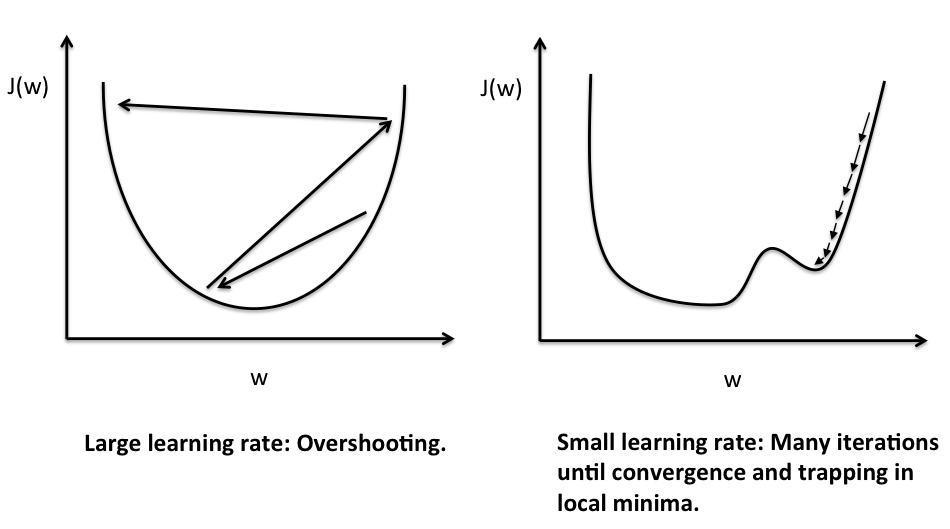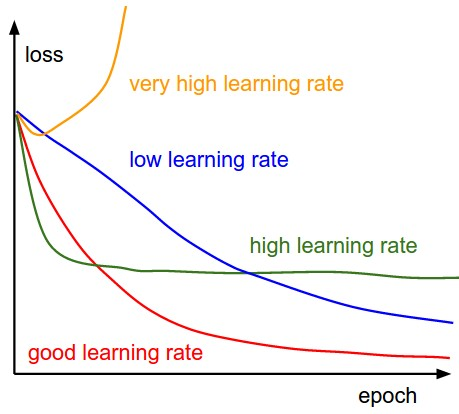# 3 Types of Gradient Descent Algorithms for Small & Large Data Sets

Introduction Gradient Descent Algorithm is an iterative algorithm to find a Global Minimum of an objective function (cost  function) J(?). The categorization of GD algorithm is for accuracy and time...

## Introduction

Gradient Descent Algorithm is an iterative algorithm to find a Global Minimum of an objective function (cost  function) J(?). The categorization of GD algorithm is for accuracy and time consuming factors that are discussed below in detail. This algorithm is widely used in machine learning for minimization of functions. Here,the algorithm to achieve objective goal of picture below is in this tutorial below.## Why use gradient descent algorithm?

We use gradient descent to minimize the functions like J(?). In gradient descent, our first step is to initialize the parameters by some value and keep changing these values till we reach the global minimum. In this algorithm, we calculate the derivative of  cost function in every iteration and update the values of parameters simultaneously using  the formula:where ‘?’ is the learning rate.

We will consider linear regression for algorithmic example in this article while talking about gradient descent, although the ideas apply to other algorithms too, such as

• Logistic regression
• Neural networks

In linear regression we have a hypothesis function:$$H(X)=\theta_0+\theta_1X_1+\ldots +\theta_nX_n$$

Where $$\theta_0,\theta_1,\ldots, \theta_n$$ are parameters and $$X_1,\ldots, X_n$$ are input features. In order to solve the model, we try to find the parameter, such that the hypothesis fits the model in the best possible way.To find the value of parameters we develop a cost function J($$\theta$$) and use gradient descent to minimize this function.

## How gradient descent algorithm works?

The following pseudo-code explains the working :

1. Initialize the parameter with some values. (Say $$\theta_1=\theta_2=\ldots =\theta_n=0$$)
2. Keep changing these values iteratively in such a way it minimize the objective function, J($$\theta$$).

## Types of Gradient Descent Algorithms

Various variants of gradient descent are defined on the basis of how we use the data to calculate derivative of cost function in gradient descent. Depending upon the amount of data used, the time complexity and accuracy of the algorithms differs with each other.## How does the batch gradient descent work?

It is the first basic type of gradient descent in which we use the complete dataset available to compute the gradient of cost function.
As we need to calculate the gradient on the whole dataset to perform just one update, batch gradient descent can be very slow and is intractable for datasets that don’t fit in memory. After initializing the parameter with arbitrary values we calculate gradient of cost function using following relation:where ‘m’ is the number of training examples.

• If you have 300,000,000 records you need to read in all the records into memory from disk because you can’t store them all in memory.
•  After calculating sigma for one iteration, we move one step.
• Then repeat for every step.
• This means it take a long time to converge.
• Especially because disk I/O is typically a system bottleneck anyway, and this will inevitably require a huge number of reads.Contour plot: after every iterationBatch gradient descent is not suitable for huge datasets. The code below explains implementing gradient descent in python.

## How does stochastic gradient descent works?

Batch Gradient Descent turns out to be a  slower algorithm. So, for faster computation, we prefer to use stochastic gradient descent.
The first step of algorithm is to randomize the whole training set. Then, for updation of every parameter we use only one training example in every iteration to compute the gradient of cost function. As it uses one training example in every iteration this algo is faster for larger data set. In SGD, one might not achieve accuracy, but the computation of results are faster.
After initializing the parameter with arbitrary values we calculate gradient of cost function using following relation:where, ‘m’ is the number of training examples

Following is the pseudo code for stochastic gradient descent:

• In the inner loop:
• Taking first step: pick first training example and update the parameter using this example, then for second example and so on
• Taking second step: pick second training example and update the parameter using this example, and so on for ‘ m ‘.
• Now take third … n steps in algorithm.
• Until we reach global minimum.SGD Never actually converges like batch gradient descent does,but ends up wandering around some region close to the global minimum.

## How does mini batch gradient descent work?

Mini batch algorithm is the most favorable and widely used algorithm that makes precise and faster results using a batch of ‘ m ‘  training examples. In mini batch algorithm rather than using  the complete data set, in every iteration we use a set of ‘m’ training examples called batch to compute the gradient of the cost function. Common mini-batch sizes range between 50 and 256, but can vary for different applications.
In this way, algorithm

• reduces the variance of the parameter updates, which can lead to more stable convergence.
• can make use of highly optimized matrix, that  makes computing of  gradient very efficient.

After initializing the parameter with arbitrary values we calculate gradient of cost function using following relation:where ‘ b ‘  is number of batches and ‘ m ‘ is number training examples.

Some of the important points to remember are:

• Updating Parameter Simultaneously-
While implementing the algorithm, updating of parameter $$\theta_1=\theta_2=\ldots =\theta_n=0$$ should be done simultaneously. This means, during values of parameters should be store first in some temporary variable then assigned to the parameters.• Learning rate ‘?’-
? is crucial parameter that controls how large steps our algorithm takes.

1. If ? is too large algorithm would take larger steps and algorithm may not converge .
2. if ? is small, then smaller will be the steps and esay to converge.• Checking working of gradient descent-
Plot the curve  between Number of Iterations and value of cost function after that number of iteration. This plot helps to identify whether gradient descent is working properly or  not.

” J(?) should decrease after every iteration and should become constant (or converge ) after some iterations.”

Above statement is because after every iteration of gradient descent and ? takes values such that J(?) moves towards depth i.e. value of J(?) decreases after every iteration.

• Variation in gradient descent with learning rate-## Summary

In this article, we learned about the basics of gradient descent algorithm and its types. These optimization algorithms are being widely used in neural networks these days. Hence, it’s important to learn. The image below shows a quick comparison in all 3 types of gradient descent algorithms: